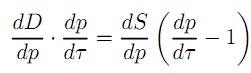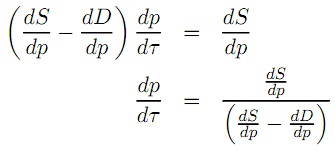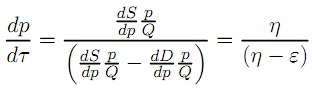#### Effects of Specific Tax on elasticity

Effects of Specific Tax on elasticity:

In the new equilibrium demand should equal supply, but demand depends on the price that consumers pay, p, supply depends on the price that suppliers receive p − τ, therefore:

D(p(τ)) = S(p(τ) − τ)

As we’ve seen before, the equilibrium price can be considered an implicit function of the environmental variable that changes, in this case τ, hence we write p(τ) to emphasize that the equilibrium price depends (indirectly) on the specific tax rate. The change of the equilibrium price due to a small change in τ is the derivative: dp/dτ and the total change in quantity demanded due to a change in price is dD/dp. By using the so called chain rule we find the total change in quantity demanded due to a small change in τ as: dD/dp . dp/dτ. On the supply side, the total change in quantity supplied due to an increase in the equilibrium price is dS/dp and the change in the price received by suppliers is [d(p(τ)−τ)]/dτ = (dp/dτ) −1, putting these changes together gives: (dS/dp) x [(dp/dτ) – 1]. From one equilibrium to another, the total change in quantity demanded should be equal to the total change in quantity supplied:Solving for the derivative dp/dτ:The demand curve is downward sloping so dD/dp < 0 and if the supply curve is upward sloping dS/dp > 0, the denominator is positive and we can conclude that the equilibrium price will increase as the specific tax is increased. If dS/dp < 0, the sign is ambiguous (could be positive or negative). By multiplying all terms in both the numerator and denominator by p/Q, we can express the derivative in terms of supply and demand elasticity:Latest technology based Economics Online Tutoring Assistance

Tutors, at the www.tutorsglobe.com, take pledge to provide full satisfaction and assurance in Intermediate Microeconomics homework help via online tutoring. Students are getting 100% satisfaction by online tutors across the globe. Here you can get homework help for Intermediate Microeconomics, project ideas and tutorials. We provide email based Intermediate Microeconomics homework help. You can join us to ask queries 24x7 with live, experienced and qualified online tutors specialized in Intermediate Microeconomics. Through Online Tutoring, you would be able to complete your homework or assignments at your home. Tutors at the TutorsGlobe are committed to provide the best quality online tutoring assistance for Economics homework help and assignment help services. They use their experience, as they have solved thousands of the Economics assignments, which may help you to solve your complex issues of Intermediate Microeconomics. TutorsGlobe assure for the best quality compliance to your homework. Compromise with quality is not in our dictionary. If we feel that we are not able to provide the homework help as per the deadline or given instruction by the student, we refund the money of the student without any delay.